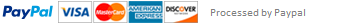### Marks Calculator in Python

Write a Python program to calculate the subject marks of a student. The input data for the program includes: the raw marks of assignment, test, tutorial exercises and exam. After receiving the inputs, the program calculates and displays the final marks of each component and total final mark. Then it decides the grade that student should get. The grade level is based on the following table according to the final total mark:

The weights of each assessment component are shown on the consol. The program should also check the validity of all the input data, including numeric input and valid range of each input data, before carrying out the calculation of marks.

Two examples of student grade calculations are shown below:

Some of the error messages that your program can display are shown below:

Assignment must be between 0 and 50.

Test mark must be between 0 and 40.

Format your output to display the marks with two decimal digits.

This assignment has been answered 6 times in private sessions.